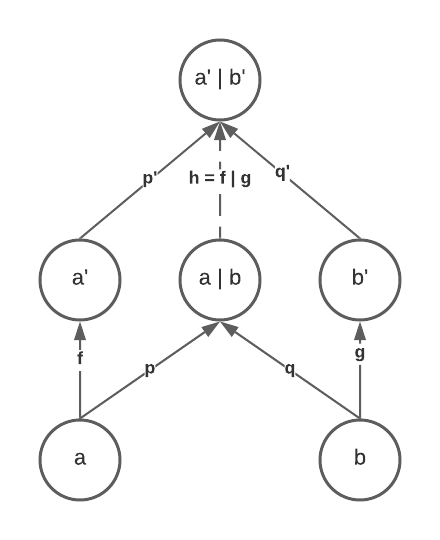## Note on Bifunctors

### From Bartosz Milewski's lecture

This is a note based on the lecture about functoriality and bifunctors. The same topic is also discussed in the Functoriality chapter from the book Category Theory for Programmers.

## What is discussed in this lecture?

This lecture discussed two main topics:

• What is a bifunctor
• product and coproduct are bifunctors, in both Hask and general categories

## What is a bifunctor?

A bifunctor maps two objects $$a$$ and $$b$$, from categories $$C$$ and $$D$$, respectively, to a new object $$e$$ in category $$E$$.

Instead of defining a new concept from scratch, we reuse the concept of functors when defining bifunctors. We could define a new category $$C \times D$$ whose objects are pairs of pairs of objects from $$C$$ and $$D$$. As a result, $$a$$ and $$b$$ form a pair $$(a, b)$$ in $$C \times D$$.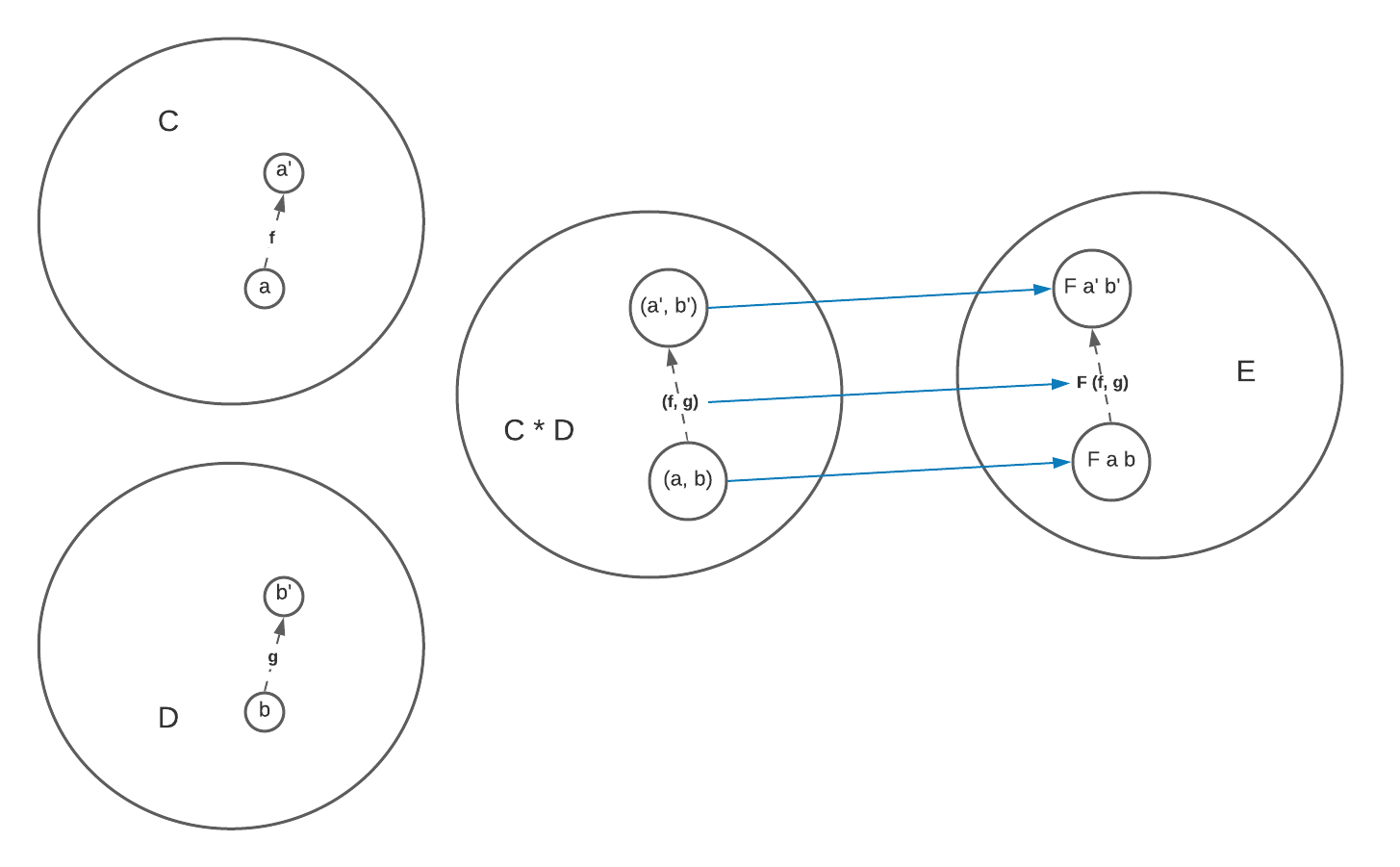A functor maps not only the objects but also the morphisms between objects. Similarly, a bifunctor should also somehow map the morphisms. Suppose we have another pair of objects $$a'$$ and $$b'$$ which are represented by an object $$(a', b')$$ in $$C \times D$$, and we have $$f$$ goes from $$a$$ to $$a'$$ and $$g$$ from $$b$$ to $$b'$$, we could thus define a new morphism called $$(f, g)$$ in $$C \times D$$ which maps the first component of $$(a, b)$$ using its first component $$f$$, and the second component using $$g$$. Note that every morphism from $$(a, b)$$ to $$(a', b')$$ is constructed by picking one morphism from the morphisms between $$a$$ and $$a'$$, and another one from the morphisms between $$b$$ and $$b'$$. Therefore, if there are $$m$$ morphisms between $$a$$ and $$a'$$, and $$n$$ between $$b$$ and $$b'$$, there will be $$m \times n$$ morphisms between $$(a, b)$$ and $$(a', b')$$.

A bifunctor, as a result, is defined as a functor $$F$$ from $$C \times D$$ to $$E$$, which maps $$(a, b)$$ to $$F a b$$ and $$(a', b')$$ to $$F a' b'$$. It also preserves the morphism structure by mapping $$(f, g)$$ to a morphism $$F (f, g)$$ between $$F a b$$ and $$F a' b'$$.

However, we usually deal with a single category when working on Hask, so actually both $$D$$ and $$E$$ are the same as $$C$$. Therefore, we could think of a bifunctor as one which takes two objects $$a$$ and $$b$$ and maps them to another object $$F a b$$. The bifunctor also maps two morphisms, $$f$$ and $$g$$, to $$F f g$$.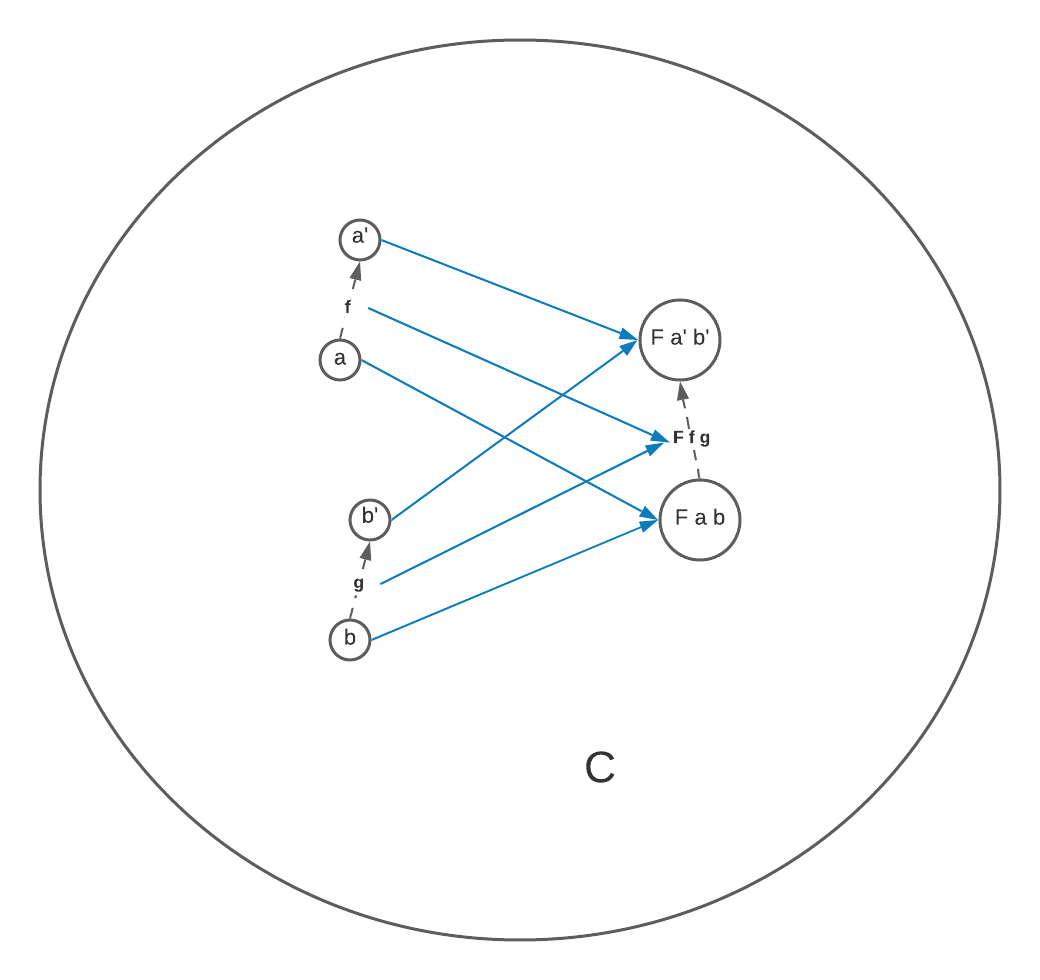## Products and coproducts are bifunctors

A product in Hask is a bifunctor which maps $$a$$ and $$b$$ to $$(a, b)$$, and $$f$$ and $$g$$ to $$(f, g)$$. Or if we define it in terms of the product category (right side), it’s just an $$id$$ mapping.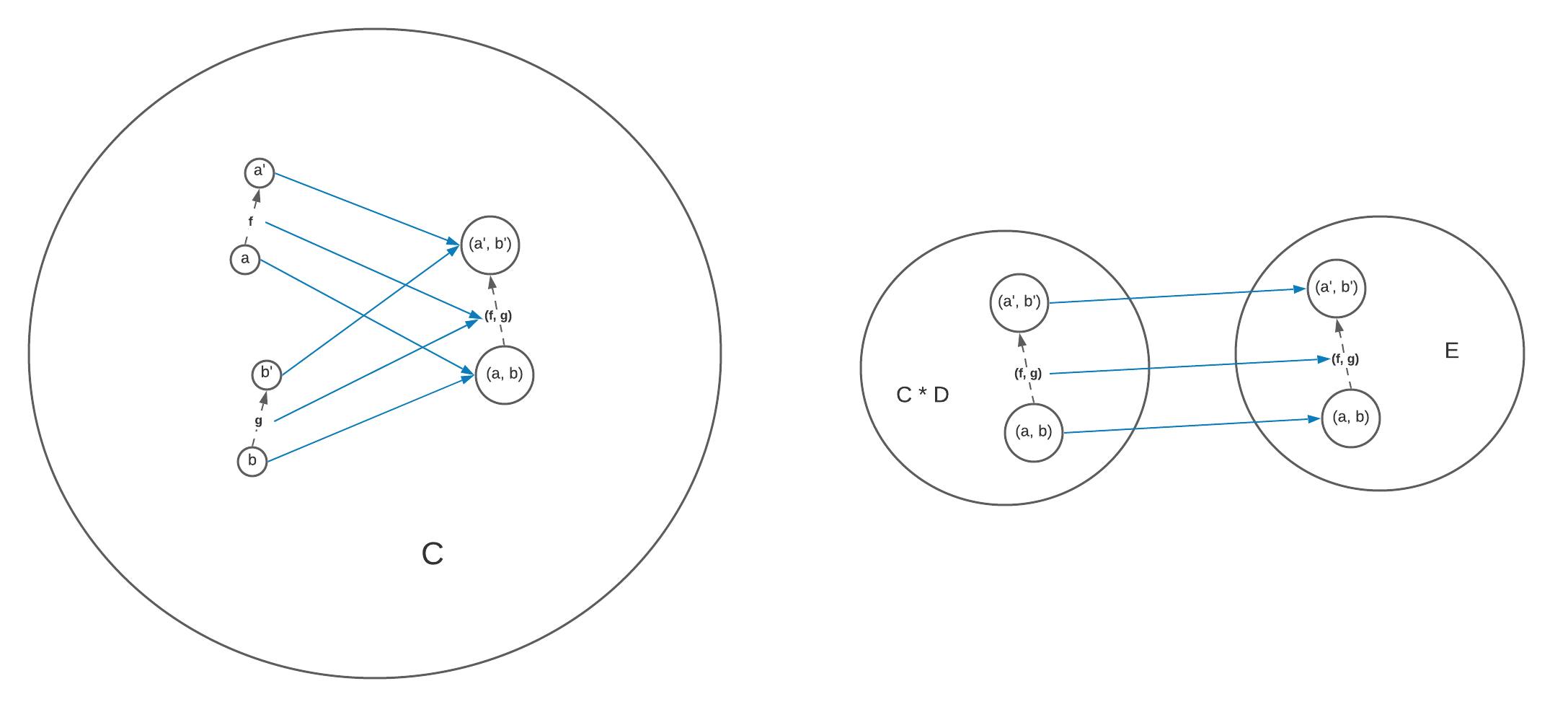Similarly, a coproduct in Hask is also a bifunctor which maps $$a$$ and $$b$$ to $$a | b$$. What is interesting is how it maps the morphisms. The coproduct bifunctor takes two morphisms $$f$$ and $$g$$, and, based on whether the source object is $$a$$ or $$b$$, apply $$f$$ or $$g$$ conditionally.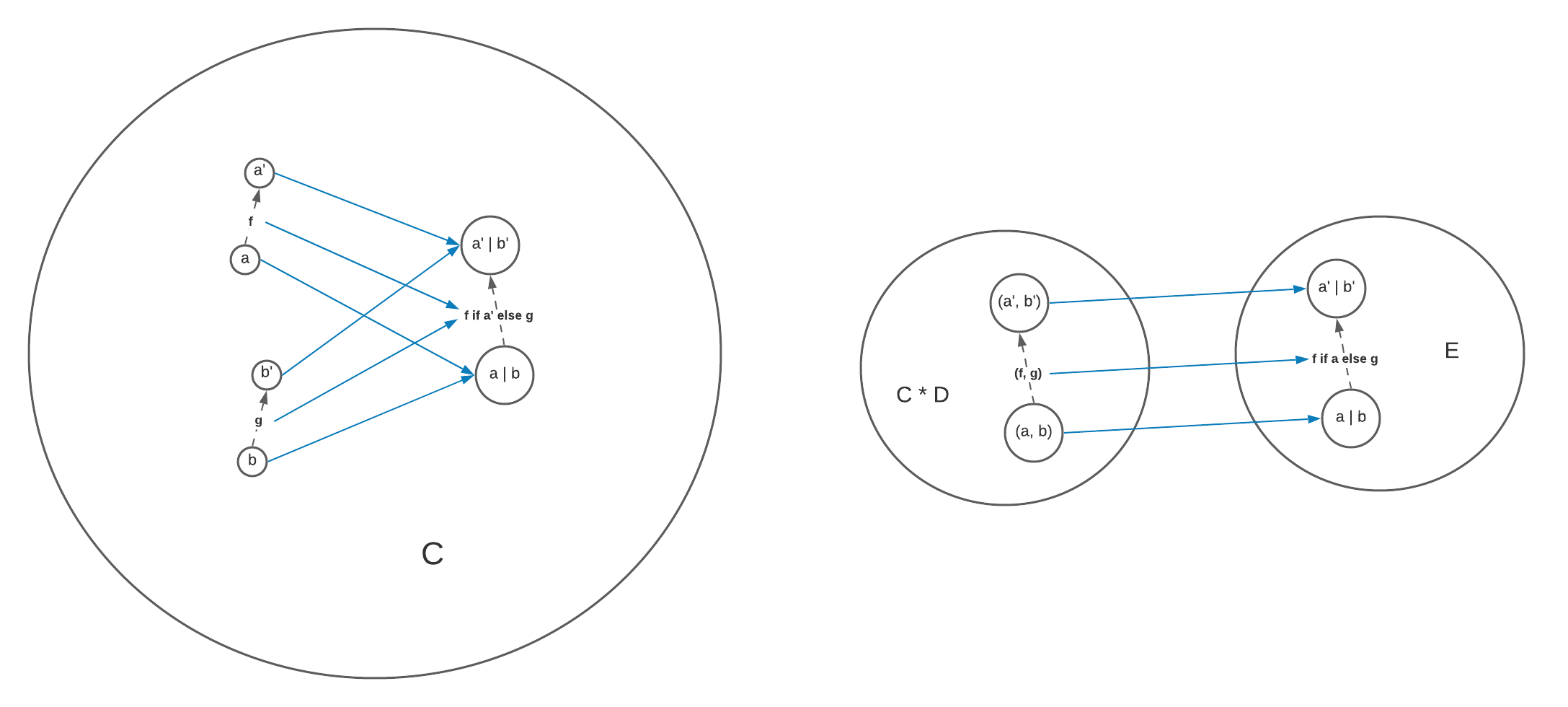### General categories

Theorem 1 (products are bifunctors) In a category where you have product defined for every pair of objects, this product is a bifunctor.

We have two objects $$a$$ and $$b$$ whose product is $$a * b$$, which means projection morphisms $$p$$ and $$q$$ from $$a * b$$ to $$a$$ and $$b$$, respectively. We also have $$a'$$, $$b'$$ and $$a' * b'$$. Now we want to map the morphisms $$f$$ and $$g$$ to some other morphism in the category which maps $$a * b$$ to $$a' * b'$$.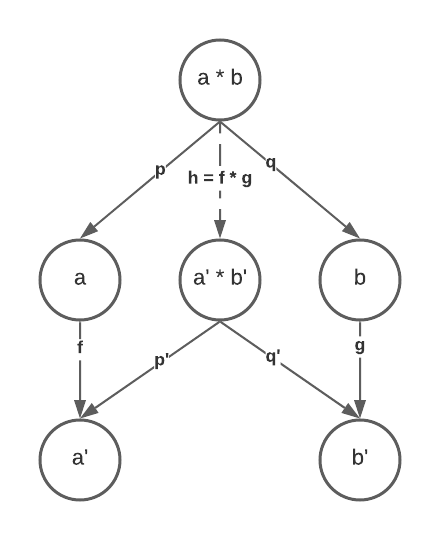We could consider $$a * b$$ to be another candidate of the product of $$a'$$ and $$b'$$. By the definition of product, there must be a unique morphism $$h$$ from $$a * b$$ to $$a' * b'$$ such that $$p' \cdot h = f \cdot p$$ and $$q' \cdot h = g \cdot q$$. Let’s define $$f * g$$ to be this $$h$$, and here is how the product bifunctor maps morphisms.

Similarly, coproducts are also bifunctors, see the figure below.